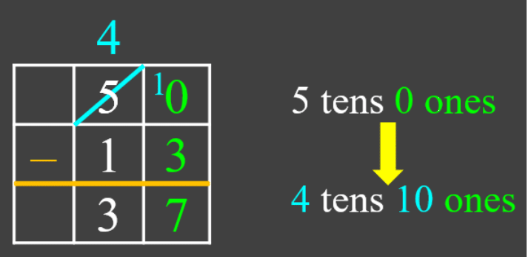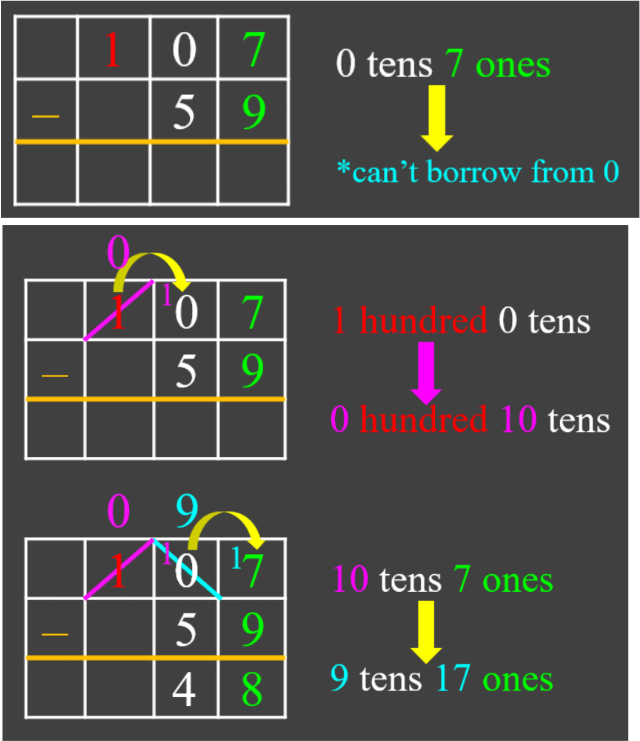# Subtracting multi-digit numbers#### All in One Place

Everything you need for better grades in university, high school and elementary.#### Learn with Ease

Made in Canada with help for all provincial curriculums, so you can study in confidence.#### Instant and Unlimited Help

0/2
##### Intros
###### Lessons
1. Introduction to Subtracting Multi-Digit Numbers:
2. Introduction to subtracting multi-digit numbers
3. Regrouping technique: borrowing when there are zeroes
0/17
##### Examples
###### Lessons
1. Subtracting 2-digit numbers with regrouping
Subtract:
1. 71 - 9
2. 50 - 13
3. 65 - 36
4. 94 - 48
2. Subtracting 3-digit numbers with regrouping
Subtract:
1. 468 - 281
2. 812 - 223
3. 950 - 671
3. Subtracting from 100 or 1000
Subtract:
1. 100 - 68
2. 100 - 49
3. 1000 - 712
4. 1000 - 543
4. Subtracting 4-, 5- and 6- digit numbers with regrouping
Subtract:
1. 5431 - 251
2. 76820 - 34951
3. 619687 - 418939
5. Subtracting three numbers
Subtract:
1. 695 - 203 - 318
2. 100 - 37 - 42
6. Subtracting multi-digit numbers with regrouping: word problem
Billy went to the electronics store with $2502. He bought a laptop for$1599. How much money does he have left?
0%
##### Practice
###### Topic Notes

In this lesson, we will learn:

• How to subtract examples with regrouping: from two 2-digit numbers up to two 6-digit numbers
• How to subtract three numbers
• How to regroup when there are zeroes

Notes:

• If you need to borrow when subtracting:
• 1 ten can be regrouped ("exchanged") for 10 ones
• 1 hundred can be regrouped for 10 tens
• 1 thousand can be regrouped for 10 hundreds
Example 1:Example 2: